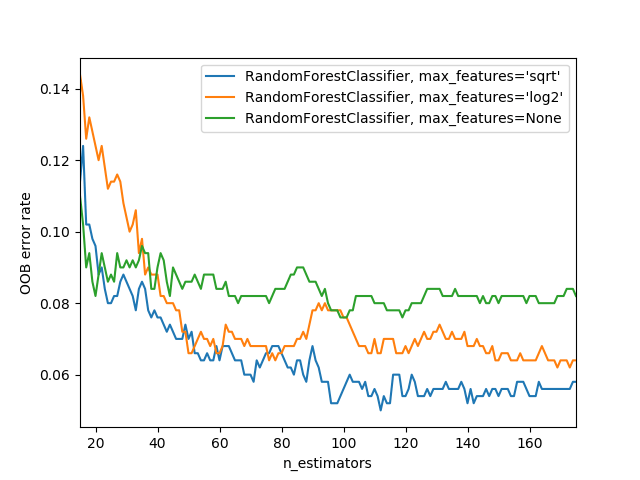/scikit-learn

# OOB Errors for Random Forests

The `RandomForestClassifier` is trained using bootstrap aggregation, where each new tree is fit from a bootstrap sample of the training observations \(z_i = (x_i, y_i)\). The out-of-bag (OOB) error is the average error for each \(z_i\) calculated using predictions from the trees that do not contain \(z_i\) in their respective bootstrap sample. This allows the `RandomForestClassifier` to be fit and validated whilst being trained .

The example below demonstrates how the OOB error can be measured at the addition of each new tree during training. The resulting plot allows a practitioner to approximate a suitable value of `n_estimators` at which the error stabilizes.

  T. Hastie, R. Tibshirani and J. Friedman, “Elements of Statistical Learning Ed. 2”, p592-593, Springer, 2009.```import matplotlib.pyplot as plt

from collections import OrderedDict
from sklearn.datasets import make_classification
from sklearn.ensemble import RandomForestClassifier, ExtraTreesClassifier

# Author: Kian Ho <[email protected]>
#         Gilles Louppe <[email protected]>
#         Andreas Mueller <[email protected]>
#
# License: BSD 3 Clause

print(__doc__)

RANDOM_STATE = 123

# Generate a binary classification dataset.
X, y = make_classification(n_samples=500, n_features=25,
n_clusters_per_class=1, n_informative=15,
random_state=RANDOM_STATE)

# NOTE: Setting the `warm_start` construction parameter to `True` disables
# support for parallelized ensembles but is necessary for tracking the OOB
# error trajectory during training.
ensemble_clfs = [
("RandomForestClassifier, max_features='sqrt'",
RandomForestClassifier(n_estimators=100,
warm_start=True, oob_score=True,
max_features="sqrt",
random_state=RANDOM_STATE)),
("RandomForestClassifier, max_features='log2'",
RandomForestClassifier(n_estimators=100,
warm_start=True, max_features='log2',
oob_score=True,
random_state=RANDOM_STATE)),
("RandomForestClassifier, max_features=None",
RandomForestClassifier(n_estimators=100,
warm_start=True, max_features=None,
oob_score=True,
random_state=RANDOM_STATE))
]

# Map a classifier name to a list of (<n_estimators>, <error rate>) pairs.
error_rate = OrderedDict((label, []) for label, _ in ensemble_clfs)

# Range of `n_estimators` values to explore.
min_estimators = 15
max_estimators = 175

for label, clf in ensemble_clfs:
for i in range(min_estimators, max_estimators + 1):
clf.set_params(n_estimators=i)
clf.fit(X, y)

# Record the OOB error for each `n_estimators=i` setting.
oob_error = 1 - clf.oob_score_
error_rate[label].append((i, oob_error))

# Generate the "OOB error rate" vs. "n_estimators" plot.
for label, clf_err in error_rate.items():
xs, ys = zip(*clf_err)
plt.plot(xs, ys, label=label)

plt.xlim(min_estimators, max_estimators)
plt.xlabel("n_estimators")
plt.ylabel("OOB error rate")
plt.legend(loc="upper right")
plt.show()
```

Total running time of the script: ( 0 minutes 10.108 seconds)

Gallery generated by Sphinx-Gallery

© 2007–2018 The scikit-learn developers
Licensed under the 3-clause BSD License.
http://scikit-learn.org/stable/auto_examples/ensemble/plot_ensemble_oob.html